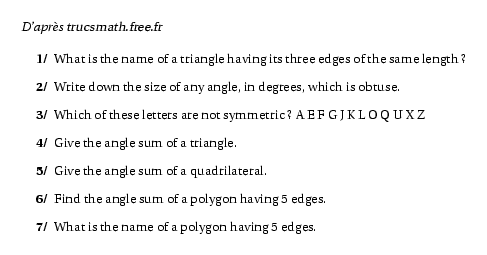Modifié le 19 Octobre 2006 à 21 h 07.Source
%@Auteur: D'après {\sl trucsmath.free.fr}\par
\selectlanguage{english}
\begin{myenumerate}
\item What is  the name of a triangle having its  three edges of the
same length ?
\item Write down the size of any angle, in degrees, which is obtuse.
\item Which of these letters are not symmetric ?   A E F G J K L O Q U
X Z
\item Give the angle sum of a triangle.
\item Give the angle sum of a quadrilateral.
\item Find the angle sum of a polygon having 5 edges.
\item What is the name of  a polygon having 5 edges.
\end{myenumerate}
\selectlanguage{frenchb}